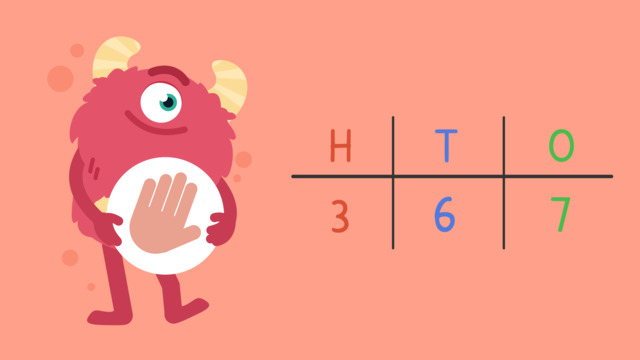# Rounding to the Nearest Hundred — Let's Practice!Rating

Be the first to give a rating!
The authorsTeam Digital
Rounding to the Nearest Hundred — Let's Practice!
CCSS.MATH.CONTENT.3.NBT.A.1

## Basics on the topicRounding to the Nearest Hundred — Let's Practice!

Today we are practicing rounding to the nearest hundred with Razzi! This video contains examples to help you further practice and grow confident in this topic.

### TranscriptRounding to the Nearest Hundred — Let's Practice!

Razzi says get these items ready, because today we're going to practice rounding to the nearest hundred. It's time to begin! Round nine hundred twenty-one to the nearest hundred. Pause the video to work on the problem, and press play when you are ready to see the solution! A two is in the tens place, so we round down. Nine hundred twenty-one rounded to the nearest hundred is nine hundred. Did you also get nine hundred? Let's tackle the next problem! Round five hundred fifty-five to the nearest hundred. Pause the video to work on the problem, and press play when you are ready to see the solution! A five is in the tens place, so we round up. Five hundred fifty-five rounded to the nearest hundred is six hundred. Did you also get six hundred? Let's tackle the final problem! Round four hundred forty-three to the nearest hundred. Pause the video to work on the problem, and press play when you are ready to see the solution! A four is in the tens place, so we round down. Four hundred forty-three rounded to the nearest hundred is four hundred. Did you also get four hundred? Razzi had so much fun practicing with you today! See you next time!

## Rounding to the Nearest Hundred — Let's Practice! exercise

Would you like to apply the knowledge you’ve learned? You can review and practice it with the tasks for the video Rounding to the Nearest Hundred — Let's Practice!.
• ### Which numbers round up, and which numbers round down?

Hints

Think about the number in the tens place. Remember, smaller values cause the number to round down.

673 rounds up to the nearest hundred, 700.

823 rounds down to the nearest hundred, 800.

Solution

0, 1, 2, 3, 4 round down.

5, 6, 7, 8, 9 round up.

• ### Find the numbers rounded to the nearest hundred.

Hints

Remember, if the tens place has a 0, 1, 2, 3, or 4, the number rounds down to the nearest hundred.

555 rounds to 600. There is a 5 in the tens place, so we round up.

545 rounds to 500. There is a 4 in the tens place, so we round down.

If the tens place has a 5, 6, 7, 8, or 9, the number rounds up to the nearest hundred.

Solution

573 rounds up to 600, because there is a 7 in the tens place.

541 rounds down to 500, because there is a 4 in the tens place.

812 rounds down to 800, because there is a 1 in the tens place.

388 rounds up to 400, because there is an 8 in the tens place.

155 rounds up to 200, because there is a 5 in the tens place.

• ### Fill in the numbers rounded to the nearest hundred.

Hints

Look at the tens place. If there is a 0, 1, 2, 3, or 4 in the tens place, the number rounds down to the nearest hundred.

For example, 942 rounds to 900.

If there is a 5, 6, 7, 8, or 9 in the tens place, the number rounds up to the nearest hundred.

For example, 889 rounds to 900.

Solution

134 rounds down to 100, because there is a 3 in the tens place.

349 rounds down to 300, because there is a 4 in the tens place.

662 rounds up to 700, because there is a 6 in the tens place.

• ### Round the numbers.

Hints

Think about the tens place. Will the number round up or down?

610 would round to 600, because there is a 1 in the tens place, so we round down to the nearest hundred.

670 would round 700 because there is a 7 in the tens place, so we round up to the nearest hundred.

Solution

710, 720, 730, and 740 all round to 700, because there is a 1, 2, 3, or 4 in the tens place.

750, 760, 770, 780, and 790 all round to 800, because there is a 5, 6, 7, 8, or 9 in the tens place.

• ### Choose the correct rounded number.

Hints

Remember, if the tens place has a 0, 1, 2, 3, or 4, we round down to the nearest hundred.

If the tens place has a 5, 6, 7, 8, or 9, we round up to the nearest hundred.

756. Think about the number in the tens place. Will 5 round up, or down?

Solution

756 rounds up to 800. Since the tens place has a 5, we round up.

• ### Round the numbers up or down to the nearest hundred.

Hints

Remember to look at the tens place to determine which way to round a number.

0, 1, 2, 3, 4 round down, 5, 6, 7, 8, 9 round up.

Solution

734 round down to 700, because there is a 3 in the tens place.

556 rounds up to 600, because there is a 5 in the tens place.

827 rounds down to 800, because there is a 2 in the tens place.

193 rounds up to 200, because there is a 9 in the tens place.

469 rounds up to 500, because there is a 6 in the tens place.